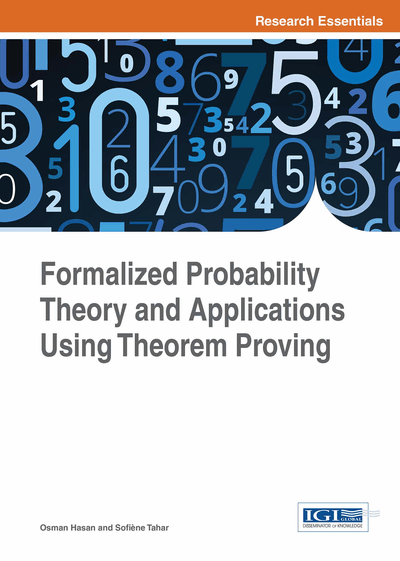# Measure Theory and Lebesgue Integration Theories

DOI: 10.4018/978-1-4666-8315-0.ch004
Available
\$37.50
No Current Special Offers

## Abstract

As discussed in the previous chapter, the fundamental theories of measure and Lebesgue integration are the prerequisites for the formalization of probability theory in higher-order logic. The scope of this chapter is primarily the formalization of these foundations. Both formalizations of measure theory and Lebesgue integral (Mhamdi, Hasan, & Tahar, 2011), presented in this chapter, are based on the extended-real numbers. This allows us to define sigma-finite and even infinite measures and handle extended-real-valued measurable functions. It also allows us to verify the properties of the Lebesgue integral and its convergence theorems for arbitrary functions. Therefore, the chapter begins with a description of the formalization of extended real numbers.
Chapter Preview
Top

## 4.1 Formalization Of Extended Real Numbers

The set of extended-real numbers R’ is the set of real numbers R extended with two additional elements, namely, the positive infinity +∞ and negative infinity -∞. Extended reals are useful in describing limiting behaviors in many mathematical fields. In particular, it is necessary to use the extended reals system to define the integration theory as otherwise the convergence theorems, like the monotone convergence and dominated convergence, would be less useful. Similarly, using extended reals for defining the measure theory allows us to define infinite measures like the sigma-finite measure. We now describe the HOL4 formalization of extended reals.

### 4.1.1 Type and Operators

An extended real is either a normal real number or positive infinity or negative infinity. We use Hol_datatype to define the new type extreal as follows:

`val _ = Hol_datatype ‘extreal = NegInf | PosInf | Normal of real ‘`

The HOL4 notation x: extreal is used to specify that the type of the variable x is extreal. The function Normal will convert a real number in HOL4 to its corresponding extended real number. For example, Normal (1: real) returns an extended real number (1: extreal). All the arithmetic operations of the real numbers need to be extended for the new type. This can be done by defining HOL4 functions over the new type and then overloading the common operators using these functions. For instance, the addition operation over the extended-real numbers can be defined using the function extreal_add as follows:

• Definition 4.1

```⊢∀ x y. extreal_add (Normal x)  (Normal y) = (Normal x + y) ˄
extreal_add (Normal _) a = a ˄
extreal_add b (Normal _)  = b ˄
extreal_add NegInf NegInf = NegInf ˄

The function is left undefined when one of the operands is PosInf and the other is NegInf. The + operator is then overloaded as

`val _ = overload_on (“+”, Term ‘extreal_add’);`

Similarly, the other arithmetic operations have also been defined for extended reals and their corresponding operators can be overloaded in HOL4 as follows:

```val _ = overload_on (“-”, Term ‘external_sub ‘);
val _ = overload_on (“*”, Term ‘external_mul ‘);
val _ = overload_on (“/”, Term ‘external_div ‘);
val _ = overload_on (“<=”, Term ‘external_le ‘);
val _ = overload_on (“<”, Term ‘external_lt ‘);
val _ = overload_on (“~”, Term ‘external_ainv ‘);
val _ = overload_on (“numeric_negate”, Term ‘external_ainv ‘);
val _ = overload_on (“inv”, Term ‘external_inv ‘);
val _ = overload_on (“abs”, Term ‘external_abs ‘);
val _ = overload_on (“logr”, Term ‘external_logr ‘);
val _ = overload_on (“lg”, Term ‘external_lg ‘);
val _ = overload_on (“exp”, Term ‘external_exp ‘);
val _ = overload_on (“pow”, Term ‘external_pow ‘);
val _ = overload_on (“sqrt”, Term ‘external_sqrt ‘);```

The order relation, for example, is extended as: ∀a ∈R’, -∞ ≤ a ≤ +∞.

• Definition 4.2

```⊢ ∀ x y.  extreal_le (Normal x) (Normal y) = (x ≤ y) ˄
extreal_le NegInf a = T ˄
extreal_le b PosInf = T ˄
extreal_le c NegInf = F ˄
extreal_le PosInf d = F ```

With the order shown in Definition 4.2, R’ is a complete lattice where every subset has a supremum and an infimum.

## Complete Chapter List

Search this Book:
Reset